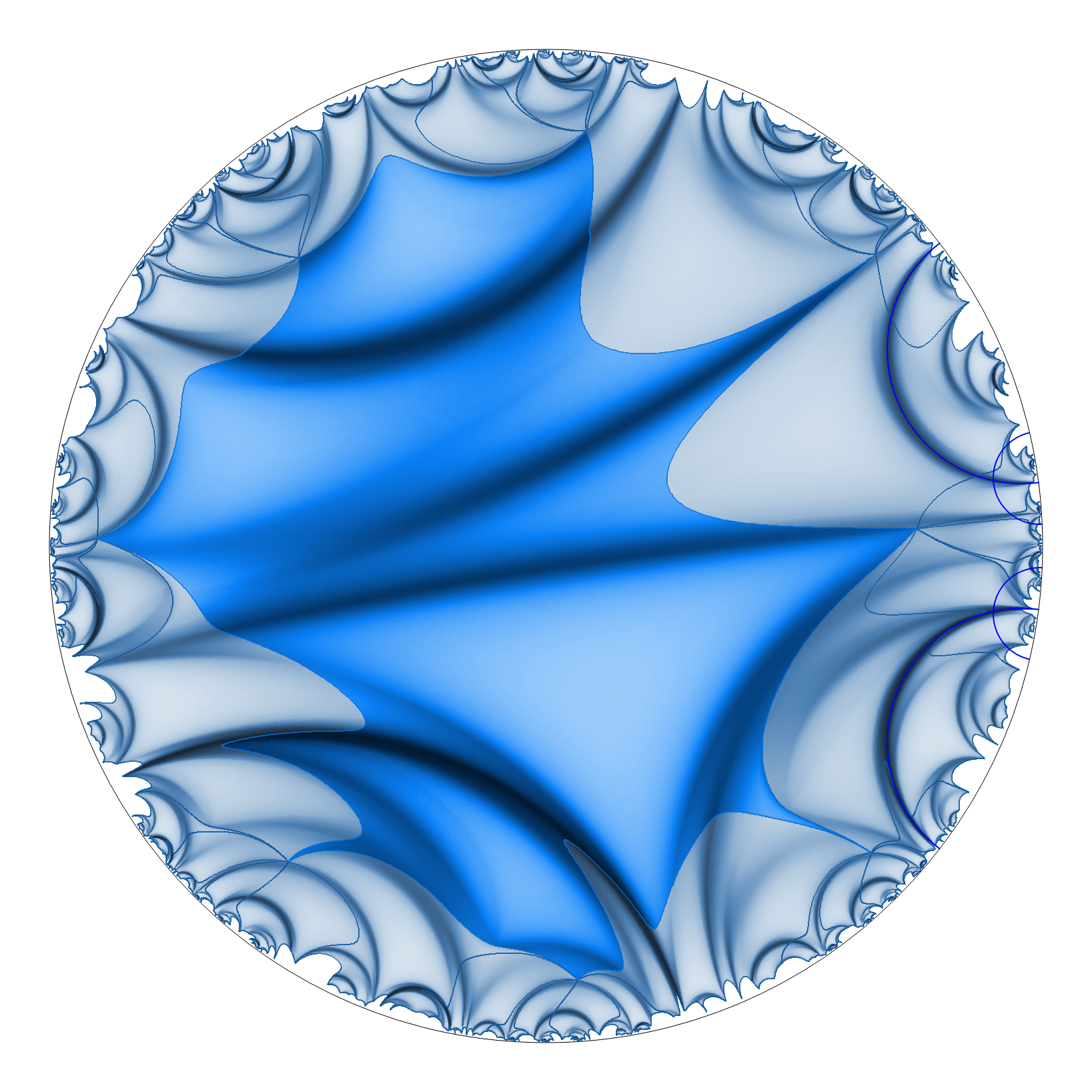Image provided by Brice Loustau (thanks!)

## A few words on my research

### A very general description

My main area of research is geometry, in particular 3-dimensional geometry and its relation to Teichm\"uller theory, the study of moduli space of complex structures on surfaces. I am attracted to elementary geometric problems, concerning for instance circle packings or polyhedra in Euclidean space, but also to deeper problems where fundamental questions in mathematics appear.

My interest in mathematics stems from the realization that many of the deepest problems in science (eg physics) or engineering boil down to mathematical questions -- the ubiquitous fact that fundamental mathematical ideas and construction, often discovered first when studying abstract-looking problems, can quickly prove powerful and even indispensable for applications in other fields of science or in technology (e.g. cryptography, coding, data analysis, etc.).

I have also from time to time studied other questions in mathematics, and also contributed to questions outside mathematics or in collaboration with scientists from other fields.

### A few contributions

Contributions to hyperbolic geometry

My first contributions to hyperbolic geometry concerned hyperbolic polyhedra (JDG 1998). The first major contribution, however, was the proof of a conjecture of Thurston (Inventiones Math. 2006): given a convex co-compact hyperbolic manifold $M$, any metric of curvature K>-1 on $\partial M$ can be uniquely realized as the induced metric on the boundary of a convex hyperbolic structure on $M$. In the same paper I also proved a dual'' statement on the third fundamental form of the boundary.

More recently, with Kirill Krasnov (Comm. Math. Phys. 2008), we introduced a new, differential-geometric way to describe the renormalized volume of hyperbolic 3-manifold and recover its main properties. This then allowed me to obtain (Math. Res. Letters 2013) a bound on the difference between the renormalized volume and the volume of a convex core of quasifuchsian manifold, as well as explicit linear bounds on those volumes in terms of the Weil-Petersson distance between the conformal structure at infinity. This last result had some impact in the field. Recently, the tools that we had developed turned out to have applications in physics (recent paper with Edward Witten, JHEP 2022).

Developing 3-dimensional anti-de Sitter (AdS) geometry and its connections to Teichm\"uller theory

Another direction of research, often in collaboration with Francesco Bonsante and sometimes with other co-authors, was the development of anti-de Sitter geometry (a Lorentzian cousin of hyperbolic geometry) and its relation to Teichm\"uller theory. Our first works in this direction (with Krasnov) introduced a notion of 3-dimensional AdS with particles'' -- cone singularities along time-like lines -- for which we then proved (with Bonsante) an analog of the Bers/Mess Double Uniformization Theorem. With Bonsante, we later used AdS geometry to prove that any quasi-symmetric homeomorphism of the circle admits a unique quasiconformal minimal Lagrangian extension to the hyperbolic plane (Inventiones math. 2010). With Jeff Danciger and Sara Maloni, we more recently used AdS geometry to answer an old question of Steiner (1832) on the possible combinatorics of polyhedra with vertices on a quadric (Inventiones math. 2020).